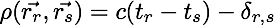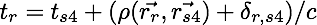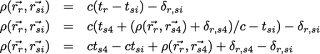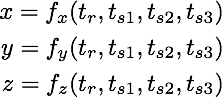Sunday, June 24, 2012

Relativity Denial: The GPS 4-Satellite Solution

I occasionally receive queries by email where a substantial response is needed.  Rather than generating an extensive response seen only in email, I try to rework it into a blog post.  This is a misconception that recently arrived in my inbox.

I have often written about how the GPS system must have relativistic corrections included in the time-of-flight computation to compute the satellite-to-receiver range needed to determining the receiver's position (see GPS, Relativity & Geocentrism and Scott Rebuttal. I. GPS & Relativity).

The minimum number of satellites needed for a position determination is three (assuming your receiver has a reasonably accurate clock), so you can determine the receiver's three position components, x,y,z in cartesian ECEF coordinates (Wikipedia).

If you have a fourth GPS satellite, then the mathematics demonstrates that the position computation can be done without the signal time from the receiver.

Misconception: If four GPS satellites are available, so that you don't need to know the time at the receiver, then relativistic corrections are not necessary.  This is evidence that relativitistic corrections are not really needed in the GPS system.

Why it is wrong: The relativistic corrections, as well as several other important corrections to the range computation, depend on the positions of the satellite(s) and the receiver.  These correction terms are in the fundamental range computation equation.  While you can use a fourth equation to eliminate the receiver time with an expression using the transmission time on the fourth satellite, the relativistic correction terms do not disappear, nor do they conveniently cancel.

Relativistic corrections remain important for accurate GPS position determination.

The Mathematical Details

The GPS Solution for Three Satellites

Using the time of the signal departing the satellite, t_s, and the time when the signal is received, t_r, we define what is called the pseudo-range, R, between the receiver, r, and the satellite, sThis is simply the distance that a radio signal, traveling at the speed of light, can travel in the time between the two clocks.  We call it a pseudo-range because it turns out that radio signal propagation between the satellite to the ground receiver is not that simple.  To deal with this, we define the true range, which I will designate with the greek letter rho, which is needed to compute the actual position of the receiverTo obtain the true range from the pseudo-range between satellite s and receiver r, a number of corrections must be applied

• Time Measurments: Errors in clocks at satellite and receiver
• Ionosphere:  A propagation delay due to the electron density in the ionosphere.  This delay is dispersive (Wikipedia) and determined by transmitting the GPS signal on two frequencies.
• Troposphere: A non-dispersive propagation correction due to radio signal refraction (Wikipedia) in troposphere.  The effect is influenced by the water content of the atmosphere.
• Tidal: A correction due to deformations of the Earth's surface due to tides (see International Earth Rotation and Reference Systems Service).
• Multipath: Timing differences created by reflection of the GPS signal from nearby objects
• Relativistic: Relativity corrections due to the motion and position of the GPS satellite as well as the motion and position of the receiver
• epsilon: General measurement errors
Each of these corrections depends on the position of the receiver and the position of the satellite.  To simplify the manipulations, here we will consolidate them into one correction, delta, which is different for each satellite, s, and receiver, r, pairing.If we want to solve the system of equations for three GPS satellites, we have:Knowing the time at the receiver, t_r, and the time of emission from each satellite, t_{s1}, t_{s2}, t_{s3}, we can solve this system of equations for the position of the receiver, \vec{r_r}, which has three components, (x,y,z), to give the position in 3-dimensional space.

The GPS solution for Four Satellites
Now suppose we have a fourth satellite which is visible to our GPS receiver.  We have a similar pseudo-range equation for it.Since we now have four equations and three unknowns, we have an overdetermined system. We can take advantage of this overdetermination to remove an input variable in the set of equations.  Since the GPS receivers tend to have the least precise clocks, we can use the fourth equation to eliminate the time of the receiver's clock from the other equations.  First, we manipulate the fourth satellite equation to give the receiver time:Once we complete solving the system, we will use this equation to determine the receiver time.  By this method, we can determine the time at the receiver to a precision higher than could be determined with the receiver clock alone.  But to solve the system, we must first substitute this result into the first three equations, where the subscript si represents the other three satellites, with i=1,2,3.Notice that all the corrections, delta, still must be included to solve the system.  In this form, we see that the correction for the receiver and satellite 4 must be added, while the corrections for the other three satellites must be subtracted.  All the corrections depend on the positions, and paths, between a given satellite and the receiver, and atop all this is a level of noise in the timing measurements.

For relativistic effects to have no impact in the four satellite configuration, the correction between the receiver and satellite 4 must exactly match the correction between the receiver and ALL of the other three satellites.  Since all four satellites are at different positions relative to the observer (otherwise they would be colliding!), the chance that these corrections have identical numerical values is small,  so relativistic corrections remain important.

Solving for x,y,z

Some might wonder how we can solve such a set of equations, where the unknown quantities are mixed in with the known quantities.  We cannot reform the equations into a clean solution of the form where all the unknowns are on the left-hand side with all the knowns on the right-hand side, such as:These interdependencies make the equations non-linear.  However, they can still be solved by iterative techniques, usually a Kalman Filter (Wikipedia).

Anonymous said...

Hi there, very nice explanation! But I'm still wondering what is the expression for d(relativistic) when you list all the corrections? Can you provide us with that expression as a function of relevant parameters? What is its order of magnitude (in average for typical parameters)? Thanking you in advance for the answers, and keep on the good effort on your blog!

W.T."Tom" Bridgman said...

Sorry for my delay. Finally completed my Balticon talk.

Probably the best paper that describes the algorithms in detail and include an example is

Marc Weiss and Neil Ashby. Gps receivers and relativity. In The 29th Annual Precise Time and Time Interval (PTTI) Applications and Planning Meeting, pages 69–84, 1998.

which is available at 29th Annual PTTI Meeting 1997.

Tom

Anonymous said...

Hi Tom,

Thanks for the explanation. However I am a little curious. In your last equation you have \del_{r,s4}-\del_{r,si}. These corrections include many effects, but it appears from your discussion these relativistic ones will cancel as long as we can assume that all the satellites are moving at the same speed and at the same altitude. Based on what I could read on wikipedia and another page on your blog, we indeed make that assumption and hence compute 38.2usec/day for all satellites.

Please don't get me wrong, I have absolute trust in SR+GR. The papers on the topic are very clear, we observe the computed relativistic effects. Furthermore they play important roles in other fields of science - many cosmic ray experiments, neutrino experiments, particle physics experiments use the GPS signals for time tagging, and not just for position determination, so the correction is indeed compulsory for these applications. And they all work.

I am not some crackpot or whatever, but based on your computation, I still cannot see why we need relativistic corrections for the GPS to achieve the neeeded accuracy. So let me put it this way: Imagine we had developed satellite technology before discovering relativity. When we run the GPS, we would observe that they satellite clocks are not running in sync with the ground clocks, and we would be very surprised. But based on the above computations, it appears that we would see all the satellite clocks to developing offsets by the same amount, so we would simply say something is going on up there, but we will use the differences anyhow to find our locations. We surely would be quite alarmed, but I don't think it would stop us from using the GPS.

What am I missing?

W.T."Tom" Bridgman said...

@Anonymous,

Ah, I see I could have made that a little clearer.

Remember that the *relative* time correction is

delta/(total time of flight)
= (difference in gravitational potential between satellite & receiver)/c^2.

The relative difference will be the same between observers and satellites if all the satellites are at the same altitude.

But to make the deltas all identically equal would require the time-of-flight of the radio signal from all satellites to the receiver be identical - all the satellites would have to be at exactly the same distance from the receiver.

While this could happen, it would be a situation which would exist for only a very short time as the satellites continued to move along their orbits.

Does that help?

Tom

Anonymous said...

Thanks for the explanation. I am not entirely convinced yet, I need to think.

The real reason why I am interested in this topic is because I am working on a short paper about relativity for a small popular science magazine. Whenever I write anything public, I try to be extremely careful, I hate quoting things without understanding the full calculation myself. I think part of the reason why xyz deniers can still "survive" despite seeing clear data for xyz, is that the people who explain things tend to oversimplify and thus the crude calculations turn into "exaggerations". When those exaggerations are identified by the deniers, they score a big goal.

For example, some time ago I had seen a documentary on BBC quoting Hawking. The documentary was claiming that were it not for the relativistic corrections, the position determined from the GPS would be erring "about 10km/day". Based on all I have read, this appears to be an "exaggeration". It seems to assume that the receiver uses his own clock so each passing day the clocks on the satellites accumulate an offset of 38usec/day.

But actually we are using the fourth satellite for extra data. Hence the full effect of 38usec/day does not really "appear" in the final calculations (as most of it seem to cancel out and we are really talking about second order effects after that cancellation). Showing this fact gives the deniers an excellent weapon - they claim we are all being "fooled by the scientists".

Hence I think it is best to properly mention that the effects we are discussing here are quite subtle - definitely essential, and in perfect agreement with GR+SR, but even if we had not discovered relativity yet, we might have still been able to make a cruder GPS. What I really want to write in my paper is how crude would that GPS really be? Would its position resolution be say err=100metres instead of something like 2m?

http://www.gps.gov/systems/gps/performance/accuracy/histogram.png

What is the real value of err? This I still don't know. If you do, I would be happy to hear.

PS: The link you provided to the 29th Annual PTTI meeting is broken. However I found another nice document by Weiss and Ashby, available here:

http://tf.boulder.nist.gov/general/pdf/1274.pdf

W.T."Tom" Bridgman said...

@Anonymous,

Consider if they launched the GPS satellites without the relativity correction (perhaps even that they didn't know about relativity). I illustrate in Relativity Denial: A response to more comments about GPS the nature of the 38 microsecond/day difference.

They would quickly find that the 3-satellite equations would not converge to a consistent solution and the error would be getting larger with time. If they realized it was a clock synchronization issue and not some other error, someone might come up with the 4-satellite solution, at which point they could use that result and solve for time at the receiver. They would find that the time at the receiver was moving steadily out of sync with the satellite clock (or the satellite clock was moving out of sync with the reciever).

The question then is would they have enough info to figure out relativity.

I've found loads of references describing relativitistic corrections for GPS on the several nanoseconds range, where 1 nanosecond corresponds to a range error of about 1 foot. There are some GPS applications used between other orbiting satellites where the claimed accuracy is on the order of centimeters.

One of the items on my ever-growing to-do list is to itemize and characterize these relativitistic corrections consistently and perhaps run a simulation illustrating the range of values. The 38 microseconds/day is the only one that accumulates over time. All the other corrections I've found oscillate over the course of the satellites in their orbits.

The link you provide looks like it might be more complete than the PTTI paper I referenced. Thanks,
Tom